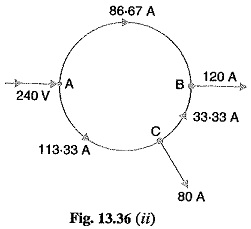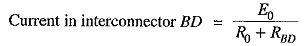## Ring Distributor:

A distributor arranged to form a closed loop and fed at one or more points is called a ring distributor. Such a distributor starts from one point, makes a loop through the area to be served, and returns to the original point. For the purpose of calculating voltage distribution, the distributor can be considered as consisting of a series of open distributors fed at both ends. The principal advantage of ring distributor is that by proper choice in the number of feeding points, great economy in copper can be affected.

The most simple case of a ring distributor is the one having only one feeding point as shown in Fig. 13.36(ii). Here A is the feeding point and tappings are taken from points B and C. For the purpose of calculations, it is equivalent to a straight distributor fed at both ends with equal voltages.### Ring Main Distributor with Interconnector:

Sometimes a ring distributor has to serve a large area. In such a case, voltage drops in the various sections of the distributor may become excessive. In order to reduce voltage drops in various sections, distant points of the distributor are joined through a conductor called interconnector. Fig.13.38 shows the ring distributor ABCDEA. The points B and D of the ring distributor are joined through an interconnector BD. There are several methods for solving such a network. However, the solution of such a network can be readily obtained by applying Thevenin’s theorem. The steps of procedure are :• Consider the interconnector BD to be disconnected [See Fig. 13.39 (i)] and find the potential difference between B and D. This gives Thevenin’s equivalent circuit voltage E0.
• Next, calculate the resistance viewed from points B and D of the network composed of distribution lines only. This gives Thevenin’s equivalent circuit series resistance R0.
• If RBD is the resistance of the inter connector BD, then Thevenin’s equivalent circuit will be as shown in Fig. 13.39(ii).Therefore, current distribution in each section and the voltage of load points can be calculated.

Scroll to Top